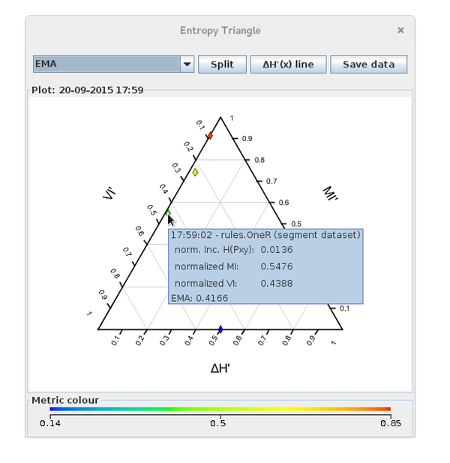A good way to configure a Weka experiment is through Java code, with only few lines of code we can setup a complete experiment. This let us play with the data modifying only the needed parts, and conserve the configuration to reproduce the experiment.

Both, the Entropy Triangle visualization and the new metrics can be used through code. The next section shows a simple example of how to add data to the Entropy Triangle, and the last section how to print an evaluation report including the package metrics.

This method requires the Java Development Toolkit (JDK) to compile the source files.

## The Entropy Triangle from code

In this example we are going to train and evaluate four classifiers with the segment dataset that is included with Weka. This dataset comes already splitted in two files for the train and test sets. Finally, we add the evaluation data to the Entropy Triangle to use it interactively.

All the code of this example goes in the same file, MyExperiment.java. We divided it in several boxes for illustration.

For a more detailed explanation on using Weka with code, see the Weka wiki pages for programmatic-use and how to use Weka in your Java code. Also, the Weka API of the developer version (3.7).

### MyExperiment.java

We create an empty text file and name it as our Java class, i.e. MyExperiment, appending .java. In this file, we are going to define a Java class with only the main method. Writing all the instructions inside the main method will make them run sequentially.

First of all, we have to create the Entropy Triangle panel, and a window, a JFrame, to place it.

import javax.swing.JFrame;

import weka.core.Instances;
import weka.core.converters.ConverterUtils.DataSource;
import weka.classifiers.evaluation.Evaluation;
import weka.etplugin.EntropyTrianglePanel;

public class MyExperiment {

public static void main(String[] args) {

JFrame frame = new JFrame();
EntropyTrianglePanel et = new EntropyTrianglePanel();

frame.setDefaultCloseOperation(JFrame.EXIT_ON_CLOSE);
frame.setVisible(true);
frame.pack();

**[NEXT SECTION CODE GOES HERE]**

}
}


### Load the dataset, train the classifier and evaluate the model

Once the Java environment is configured, we can start with the Weka instructions.

Some Weka methods throw Java exceptions if something does not go as expected. Therefore, we will surround our code in a try block with a basic catch to print the error trace.

First, we have to load the train and test sets of instances. We set the index of the class attribute to the last one, we can skip this if the arff files already has defined the class attribute.

Once we have the data loaded we are going to test it with a ZeroR classifier to have a baseline. The procedure is as follows:

1. Create an Evaluation object initialized with the prior probabilities of the train dataset.
2. Create a ZeroR classifier object. We use the fully qualified name for classifiers to avoid handling imports when changing of classifiers, but you can use the class name and import it as well.
3. Train the classifier with the train dataset.
4. Evaluate the classifier for the test dataset.
try {
DataSource source = new DataSource("./datasets/segment-challenge.arff");
Instances train = source.getDataSet();
train.setClassIndex(train.numAttributes() - 1);
test.setClassIndex(test.numAttributes() - 1);

Evaluation eval = new Evaluation(train);
weka.classifiers.rules.ZeroR zr = new weka.classifiers.rules.ZeroR();
zr.buildClassifier(train);
eval.evaluateModel(zr, test);

**[NEXT SECTION CODE GOES HERE]**

} catch (Exception e) {
System.out.println("Error on main");
e.printStackTrace();
}


Now, we have the results stored in the Evaluation object and ready to be added to the Entropy Triangle.

### Add data to the plot

The manager of the Entropy Triangle data is the EntropyTrianglePanel object we defined previously.

To add evaluation data to the visualization we only have to call the EntropyTrianglePanel addData() method.

The Evaluation object and the classifier are passed as the first two arguments. The third argument is a string used to identify the dataset, we use the dataset relation name for consistency. The last argument is another string used for timestamp information; the experiment execution timestamp is used if the argument is null.

  et.addData(eval, zr, test.relationName(), null);

**[NEXT SECTION CODE GOES HERE]**


The main advantage of the Entropy Triangle is that lets you compare easily different dataset-classifier setups. We can proceed similarly to add the evaluation of other classifiers, or try different datasets, either loading different arff files or applying Weka preprocessing filters to the Instances objects.

In this experiment, we are going to compare different classifiers with the same dataset. For that, we repeat the instructions we used before for the ZeroR classifier. The train and test Instances objects are used only for reading the instances, so can be safely reused. The Evaluation object, eval, is overwritten with new object that only has the train dataset prior probabilities.

We are going to test the OneR, NaiveBayes, and J48 (C4.5) Weka classifiers with the default options.

  eval = new Evaluation(train);
weka.classifiers.rules.OneR oner = new weka.classifiers.rules.OneR();
oner.buildClassifier(train);
eval.evaluateModel(oner, test);

eval = new Evaluation(train);
weka.classifiers.bayes.NaiveBayes nb = new weka.classifiers.bayes.NaiveBayes();
nb.buildClassifier(train);
eval.evaluateModel(nb, test);

weka.classifiers.trees.J48 j48 = new weka.classifiers.trees.J48();
j48.buildClassifier(train);
eval = new Evaluation(train);
eval.evaluateModel(j48, test);


### Running the experiment

To compile and run the experiment we have to include in the classpath the weka.jar and EntropyTriangle.jar files. We can append the -classpath option to the javac and java commands or append the files to the classpath variable of the terminal session.

#### Setting the classpath

• Linux / Mac

$export CLASSPATH=${CLASSPATH}:<path-to>/weka.jar
$export CLASSPATH=${CLASSPATH}:${HOME}/wekafiles/packages/EntropyTriangle/EntropyTriangle.jar  • Windows > set CLASSPATH=%CLASSPATH%;.;%PROGRAMFILES%/Weka-3-7/weka.jar > set CLASSPATH=%CLASSPATH%;%USERPROFILE%/wekafiles/packages/EntropyTriangle/EntropyTriangle.jar  #### Compile and run  # Compile the experiment$ javac MyExperiment.java

# Run!!
\$ java MyExperiment


Running the program opens the Entropy Triangle window with the evaluation results. Now they can be explored interactively.## Printing the plugin metrics

The plugin metrics are integrated in the Weka Evaluation class. This makes them available on all the interfaces, including the output of our code.

To print a classification report, like in the Weka explorer, we have to call the method toSummaryString() of the evaluation object.

If we want to include the information-theoretic statistics in the report, we have to call the method with true as argument: toSummaryString(true).